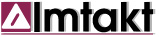﻿ IMTAKT HPLC TOOLSIMTAKT GLOBAL NETWORK

IMTAKT HPLC TOOLS
7   Frational Inch Converter NEW
The HPLC column tubings and the like use fractional inches. This tool is for converting between units of inches, fractional inches, and millimeters.

The sample injection volume or loading amount for an HPLC column is generally proportional to the column's cross-sectional area.
This tool can easily calculate the target column's loading volume or amount when the column's internal diameter is changed.

5 Peak Separation Simulator
MENU 1: Input tR1, tR2, and N. Calculated Rs.
MENU 2: Input tR1, tR2, and Rs. Calculated N.
MENU 3: Input tR1 and tR2. Calculated N to get Rs=1.5.
MENU 4: Input tR1 and N. Calculated tR2 to get Rs=1.5.

4 Solution Dilution Calculator
This calculator is a tool for an accurate solution dilution.
Input an initial concentration value/unit, initial or final volume, select unit you want to convert to, then press “CALCULATE”.
Molar weight is required to convert between % concentration and molar concentration.
Solution density is assumed to be "1".

3 Concentration Unit Converter
This is a concentration unit converter tool for accurate solution preparation.
Input your starting concentration value/unit and select the desired output unit to get the final concentration value.
Mass concentration can easily be converted from a % value to molar mass.
For very low concentrations or if a number is not entered, a density value of “1” will be automatically assigned.

2 Sample Concentration Calculator
Accurate weighing of analytes is an essential task when preparing your sample solution for injection.
This tool will make the tedious process of calculating sample weight and solution volume much easier to achieve a desired sample concentration.
This tool will also make it simple to find the molar concentration of your sample by inputting the molar mass (molecular weight).

1 Column Pressure Unit Converter
HPLC instruments use a wide variety of pressure units, even though SI has defined the standard unit of measuring pressure to be “MPa”.
Some common units used are MPa, bar, psi and kgf/cm2.
This tool makes it convenient to convert a pressure value from one unit to another.

 YAZAWA Itaru (Imtakt Corp., JAPAN) [JAZZ1959]